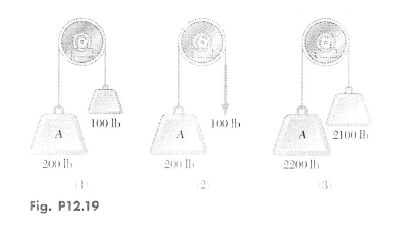# Problem: Each of the systems shown is initially at rest. Neglecting axle friction and the masses of the pulleys, determine for each system:(a) the acceleration of block A(b) the velocity of block A after it has moved through 10 ft.(c) the time required for block A to reach a velocity of 20 ft/s.

###### FREE Expert Solution

In this problem, we're going to use Newton's second law of motion, which states:

$\overline{){\mathbf{\sum }}{\mathbf{F}}{\mathbf{=}}{\mathbf{m}}{\mathbf{a}}}$, where ΣF is the sum of forces, m is the mass, and a is the acceleration.

We also need to get m in our case.

$\overline{){\mathbf{m}}{\mathbf{=}}\frac{\mathbf{W}}{\mathbf{g}}}$, where W is weight and g is the acceleration due to gravity.

System 1

(a)

Taking Newton's equation on block A:

$\begin{array}{rcl}\mathbf{\sum }{\mathbf{F}}_{\mathbf{y}}& \mathbf{=}& {\mathbf{m}}_{\mathbf{A}}{\mathbf{a}}_{\mathbf{A}}\\ {\mathbf{W}}_{\mathbf{A}}\mathbf{-}\mathbf{T}& \mathbf{=}& \frac{{\mathbf{W}}_{\mathbf{A}}}{\mathbf{g}}{\mathbf{a}}_{\mathbf{A}}\\ \mathbf{T}& \mathbf{=}& {\mathbf{W}}_{\mathbf{A}}\mathbf{-}\frac{{\mathbf{W}}_{\mathbf{A}}}{\mathbf{g}}{\mathbf{a}}_{\mathbf{A}}\\ & \mathbf{=}& \mathbf{200}\mathbf{-}\frac{\mathbf{200}}{\mathbf{g}}{\mathbf{a}}_{\mathbf{A}}\end{array}$

T = 200(1 - aA/g) ........................... (1)

Now, consider block B:

$\begin{array}{rcl}\mathbf{\sum }{\mathbf{F}}_{\mathbf{y}}& \mathbf{=}& {\mathbf{m}}_{\mathbf{B}}{\mathbf{a}}_{\mathbf{B}}\\ \mathbf{T}\mathbf{-}{\mathbf{W}}_{\mathbf{B}}& \mathbf{=}& \frac{{\mathbf{W}}_{\mathbf{B}}}{\mathbf{g}}{\mathbf{a}}_{\mathbf{B}}\\ \mathbf{T}& \mathbf{=}& {\mathbf{W}}_{\mathbf{B}}\mathbf{+}\frac{{\mathbf{W}}_{\mathbf{B}}}{\mathbf{g}}{\mathbf{a}}_{\mathbf{B}}\\ & \mathbf{=}& \mathbf{100}\mathbf{+}\frac{\mathbf{100}}{\mathbf{g}}{\mathbf{a}}_{\mathbf{B}}\end{array}$

T = 100(1 + aB/g) ................. (2)

Let's equate equations (1) and (2) and remember that |aA| = |aB|.

96% (41 ratings)###### Problem Details

Each of the systems shown is initially at rest. Neglecting axle friction and the masses of the pulleys, determine for each system:

(a) the acceleration of block A

(b) the velocity of block A after it has moved through 10 ft.

(c) the time required for block A to reach a velocity of 20 ft/s.